• correlation
• similarity measures
• plotting similarity

This session is based on chapter 6 of Levshina’s How to do linguistics with R. One of the aims of this course is that you should be able to go into any chapter of this textbook and follow the methods

Install the `Rling` package from https://benjamins.com/sites/z.195/content/package.html (or search Rling download).

``library(tidyverse)``
``````package ‘tidyverse’ was built under R version 3.3.2Loading tidyverse: ggplot2
package ‘ggplot2’ was built under R version 3.3.2package ‘readr’ was built under R version 3.3.2package ‘purrr’ was built under R version 3.3.2package ‘dplyr’ was built under R version 3.3.2Conflicts with tidy packages ------------------------------------------------------------------------------------------------
filter(): dplyr, stats
lag():    dplyr, stats``````
``````library(Rling)
data(ldt) # lexical decision time data``````

Check out the data:

``head(ldt)``

Plot word length against reaction time.

``ldt %>% ggplot(aes(x=Length, y=Mean_RT)) + geom_point() + geom_smooth(method="lm")``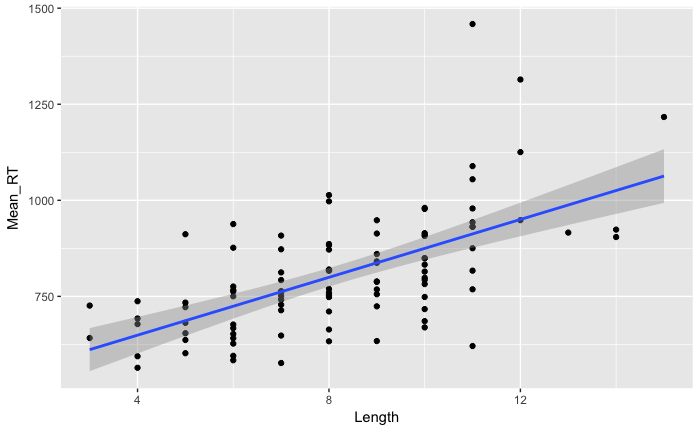Intro to linear regression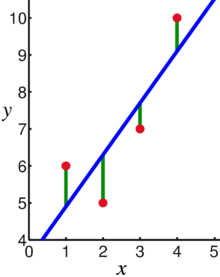Linear regression assumes that the observations (red points) show an underlying relationship between x and y (the blue line), which is obscured by some noise/random/other factors (green lines, called residuals). The residuals are the deviations from perfect correlation.

To calculate the linear model without plotting it, use the lm function:

`r`r m <- lm(Mean_RT ~ Length, data=ldt)

This contains all the data. You can look at the residuals:

``````#residuals(m) %>% head()
``````    marveled   persuaders      midmost       crutch resuspension efflorescent
19.595      102.747      146.269       41.993      175.249       -1.841 ``````

Or plot them (use a histogram)

``tibble(residuals=residuals(m)) %>% ggplot(aes(x=residuals)) + geom_histogram(bins=50)``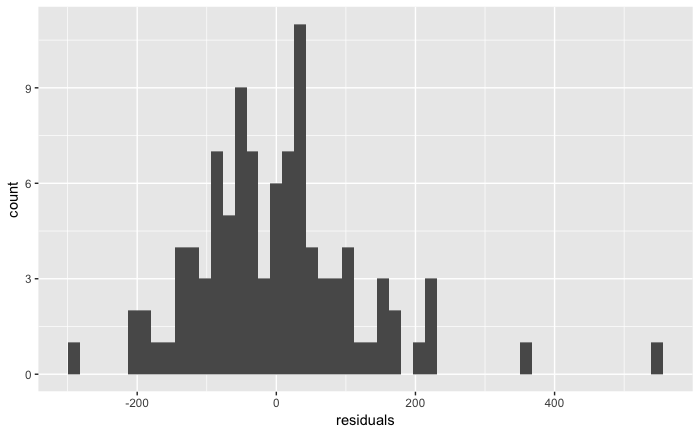Measure the correlation (using least squares, minimising the squares of the residuals)

``cor(ldt\$Mean_RT, ldt\$Length)``
`` 0.6147``
``````#help(cor)
#cor(Mean_RT, Length, data=ldt)``````

Test the strength of this correlation. Basically, is zero within the 95% confidence interval?

``cor.test(ldt\$Mean_RT, ldt\$Length, alternative="greater")``
``````
Pearson's product-moment correlation

data:  ldt\$Mean_RT and ldt\$Length
t = 7.7, df = 98, p-value = 5e-12
alternative hypothesis: true correlation is greater than 0
95 percent confidence interval:
0.5001 1.0000
sample estimates:
cor
0.6147 ``````

Very high probability that the true correlation is greater than zero.

Compare with some random data:

``````ran.data <- tibble(a=rnorm(20, 10, 1), b=rnorm(20, 10, 1))
ran.data %>% ggplot(aes(x=a, y=b)) + geom_point() + geom_smooth(method="lm")``````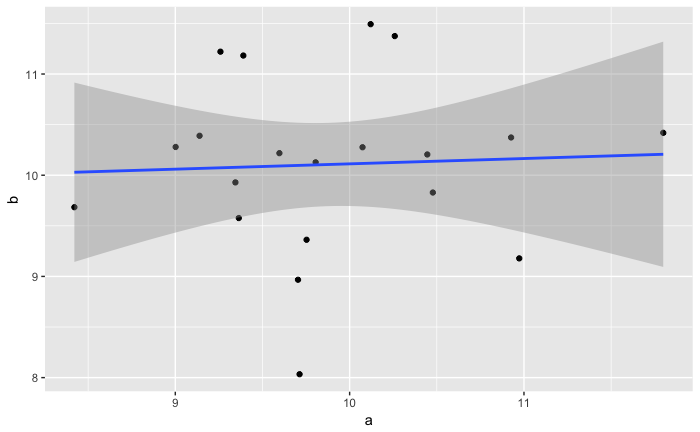There might seem to be a correlation:

``````
Pearson's product-moment correlation

data:  ran.data\$a and ran.data\$b
t = 0.46, df = 8, p-value = 0.7
alternative hypothesis: true correlation is not equal to 0
95 percent confidence interval:
-0.5214  0.7179
sample estimates:
cor
0.1611 ``````

But do we believe this? (use two-sided alternative: testing that the value is not zero)

``cor.test(ran.data\$a, ran.data\$b, alternative="two.sided")``
``````
Pearson's product-moment correlation

data:  ran.data\$a and ran.data\$b
t = 0.21, df = 18, p-value = 0.8
alternative hypothesis: true correlation is not equal to 0
95 percent confidence interval:
-0.4028  0.4805
sample estimates:
cor
0.04828 ``````

(at the p-value cut-off of 0.05, one in twenty times this demo won’t work)

``````ran.data <- tibble(a=runif(100, 0, 20))
ran.data\$b <- ran.data\$a + rnorm(100, 0, 10)
``ran.data %>% ggplot(aes(x=a, y=b)) + geom_point() + geom_smooth(method="lm")``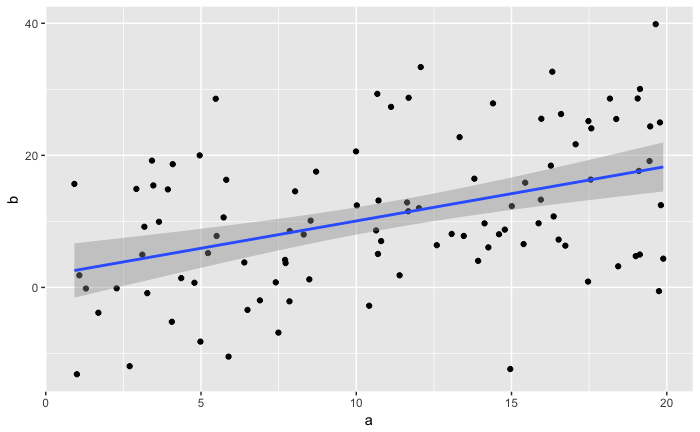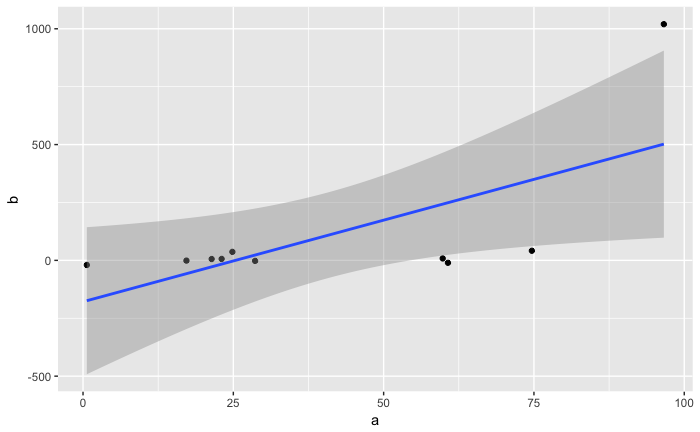Discussion point:

• don’t confuse effect size with statistical significance!

## Outliers

• False correlations with outliers

``````ran.data <- tibble(a=runif(20, 0, 10), b=runif(20, 0, 10))
ran.data %>%
arrange(desc(a)) %>%
mutate(rank=1:n()) %>%
mutate(b=if_else(rank < 4, b+100, b)) -> ran.data.2
ran.data.2 %>% ggplot(aes(x=a, y=b)) + geom_point() + geom_smooth(method="lm")``````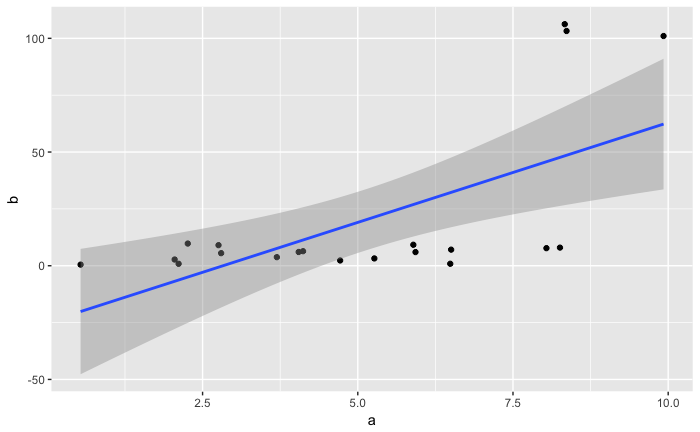``cor(ran.data.2\$a, ran.data.2\$b)``
`` 0.6366``
``cor.test(ran.data.2\$a, ran.data.2\$b, alternative="two.sided")``
``````
Pearson's product-moment correlation

data:  ran.data.2\$a and ran.data.2\$b
t = 3.5, df = 18, p-value = 0.003
alternative hypothesis: true correlation is not equal to 0
95 percent confidence interval:
0.2702 0.8420
sample estimates:
cor
0.6366 ``````
``````Parsed with column specification:
cols(
wals.code = col_character(),
name = col_character(),
family = col_character(),
F82A = col_integer(),
F83A = col_integer(),
F85A = col_integer(),
F86A = col_integer(),
F87A = col_integer(),
F88A = col_integer(),
F89A = col_integer(),
F90A = col_integer()
)``````

`r`r head(wals)

Solution: remove outliers

• Check ldt data for outliers in mean_RT
• What might these mean?
• Remove outliers and recalculate correlation

## Assumptions of Pearson correlation

• The sample is randomly selected from the population it represents
• Both variables are interval-scaled
• The sample size is large (> 30); or for smaller samples, both variables come from a bivariate normal distribution. This means: both variables are normally distributed, and their linear combination is also normally distributed.
• The residual variance is homoscedastic). This means: the relationship between the variables is of equal strength across the entire range of both variables
• The residuals are independent (no autocorrelation)

## Distance

From here on is for Session #8

``wals <- read_tsv("./typ/wals_data.tsv")``
``````Parsed with column specification:
cols(
wals.code = col_character(),
name = col_character(),
family = col_character(),
F82A = col_integer(),
F83A = col_integer(),
F85A = col_integer(),
F86A = col_integer(),
F87A = col_integer(),
F88A = col_integer(),
F89A = col_integer(),
F90A = col_integer()
)``````
``head(wals)``

Check the data quality. How much is missing?

``````wals %>% group_by(wals.code) %>%
mutate(N=sum(!is.na(F82A), !is.na(F83A), !is.na(F85A), !is.na(F86A), !is.na(F87A), !is.na(F88A), !is.na(F89A), !is.na(F90A))) %>%
ggplot(aes(x=N)) + geom_histogram(bins=10)``````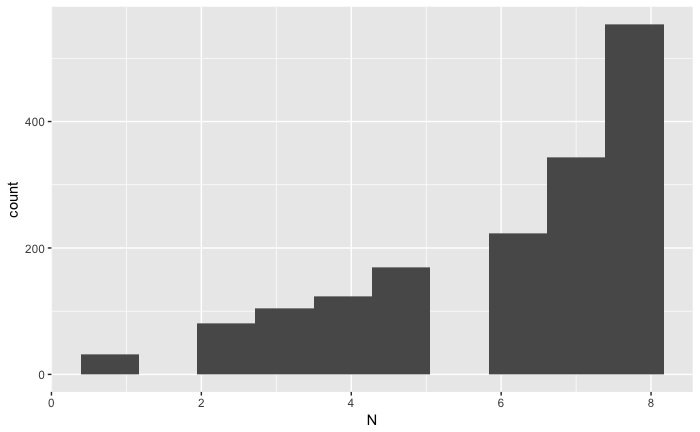Let’s just take the complete samples

``````wals %>% group_by(wals.code) %>%
mutate(N=sum(!is.na(F82A), !is.na(F83A), !is.na(F85A), !is.na(F86A), !is.na(F87A), !is.na(F88A), !is.na(F89A), !is.na(F90A))) %>%
filter(N==8) -> wals.554``````

554 rows.

Now let’s make a distance matrix. We start from an empty matrix, then fill it pair by pair

`r`r biplot(prcomp(as.dist(m)))